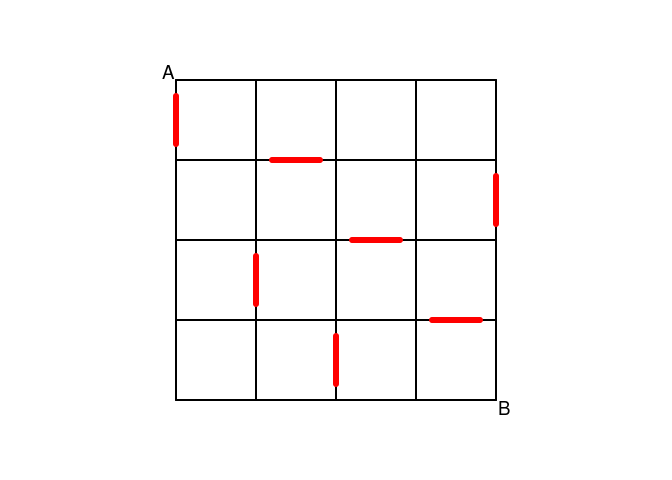# Introduction

This puzzle was published in New Scientist in June 20201. It is a practical example of a problem in graph theory. This vignette explains how the puzzle can be solved with redecison.

# The puzzle

Three friends agree to drive from A to B via the shortest road possible (driving down or right at all times). They are hungry, so also want to drive through a Big Burger restaurant, marked in red. They are arguing about how many shortest routes will pass through exactly one Big Burger. Xenia: “I reckon there are 10.” Yolanda: “I’d say more like 20.” Zara: “No you’re both wrong, I bet there are more than 50.” Who is right, or closest to right?# Constructing the graph

The grid has 25 nodes and 40 edges (20 horizontal and 20 vertical). These form a directed graph because it is allowed to drive down or right only. Seven of the edges are defined as “Big Burger” edges. Because it is not possible to find a path from any node which revisits that node, the graph is acyclic (a directed acyclic graph, DAG).

Although it possible to construct the graph by creating 25 node objects explicitly, it is more compact to create a list of vertices in a loop construct. Indices $$i = [1 .. 5]$$ and $$j = [1 .. 5]$$ are used to identify grid intersections in the vertical and horizontal directions respectively. Each node is labelled as $$N_{i,j}$$ and the index of node $$N_{i,j}$$ in the list is $$5(i-1)+j$$.

Similarly, the 40 edges (arrows) are constructed more compactly in a list, with horizontal edges being labelled $$H_{i,j}$$ (the horizontal edge joining node $$N_{i,j}$$ to node $$N_{i,j+1}$$) and the vertical edges similarly as $$V_{i,j}$$.

# create vertices
V <- list()
for (i in 1:5) {
for (j in 1:5) {
V <- c(V, Node$new(paste("N",i,j,sep=""))) } } # create edges E <- list() for (i in 1:5) { for (j in 1:4) { E <- c(E, Arrow$new(V[[5*(i-1)+j]], V[[5*(i-1)+j+1]], paste("H",i,j,sep="")))
}
}
for (i in 1:4) {
for (j in 1:5) {
E <- c(E, Arrow$new(V[[5*(i-1)+j]], V[[5*i+j]], paste("V",i,j,sep=""))) } } # create graph G <- Digraph$new(V,E)

# Finding the paths

Method paths finds all possible paths between any two nodes, where a path is defined as a sequence of distinct and adjacent nodes. Because the restaurants are specific edges, each path is converted to a walk, which is a path defined as sequence of connected, non-repeating edges.

In this case, the number of restaurants traversed by each path is counted by comparing the label associated with each edge in each path with the labels of the edges which contain a restaurant.

# get all paths from A to B
A <- V[]
B <- V[]
P <- G$paths(A,B) # convert paths to walks W <- lapply(P,function(p){G$walk(p)})
# count and tabulate how many special edges each walk traverses
BB <- c("V11", "H22", "V25", "H33", "V32", "H44", "V43")
nw <- sapply(W, function(w) {
lv <- sapply(w, function(e) {e\$label() %in% BB})
return(sum(lv))
})
# tabulate
ct <- as.data.frame(table(nw))

# Solution found by rdecision

The number of paths which pass through exactly one Big Burger is 23. In total there are 70 paths from A to B, with the number of restaurants $$n$$, traversed by each path as follows:

n frequency
0 6
1 23
2 27
3 13
4 1

# Provided solution

Yolanda’s estimate is closest - there are 23 shortest routes from A to B that pass through exactly one Big Burger. One way to solve this kind of puzzle is to systematically work from A and keep track of how many ways there are of reaching each point. With this problem, you should keep a separate count of how many ways there are of reaching each point after (a) zero or (b) one Big Burger visits. For line segments that contain a Big Burger, (b) becomes equal to (a) then becomes equal to 0 with the old value for (b) effectively discarded.